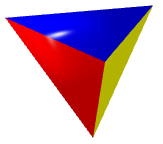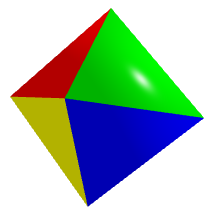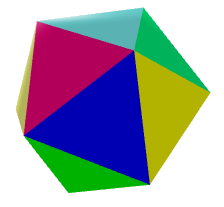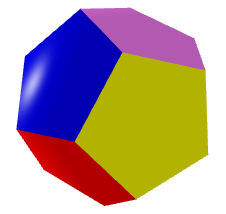# Platonic Solids

There are only five polyhedra that can be made using a regular polygon as their faces and having the same number of this polygons meet at each vertex. The five Platonic Solids are the tetrahedron, cube, octahedron, icosahedron and dodecahedron (Figure 1).

TETRAHEDRON CUBE OCTAHEDRON ICOSAHEDRON DODECAHEDRONFigure 1: The Platonic Solids. Click to see a 3D Model that you can zoom and rotate.

The following table describes the main properties of the Platonic Solids. The Dual of a solid is the polyhedron obtained joining the centers of adjacent faces; therefore, the dual has the number of vertices and edges interchanged. The Three-dimensional Constructive Coefficient gives an idea of the complexity of a solid.

PLATONIC SOLID PICTURE NUMBER OF FACES FACES PER VERTEX VERTICES EDGES THREE-DIMENSIONAL COSNTRUCTIVE COEFFICIENT DUAL SOLID PICTURE

TETRAHEDRON4 3 4 6 1 TETRAHEDRONCUBE6 3 8 12 2 OCTAHEDRONOCTAHEDRON8 4 6 12 2 CUBEICOSAHEDRON20 5 12 30 5 DODECAHEDRONDODECAHEDRON12 3 20 30 5 ICOSAHEDRONTable 1: Main properties of the Platonic Solids